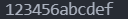#DAY 14
0

## Day 14 : 基礎套件的介紹-os套件，幫助你管理資料

``````import os
``````

``````import os
os.mkdir('./test')
``````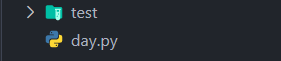``````import os
os.rmdir('./test')
``````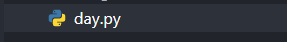``````import os
list = os.listdir('./test')
print(list)
``````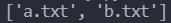open中可以放三個參數，分別是檔案路徑以及讀寫方式，還有編碼方式。我們先在a.txt裡面打入123456並把python程式碼改成這樣:

``````import os
f = open( "./test/a.txt", 'r', encoding='utf-8')
``````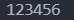r : 單純讀取這個檔案內的資料，不能做修改。

w : 更改這個檔案。

a : 再不引響檔案的狀況下，在檔案最後方追加資料。

``````import os
f = open( "./test/a.txt", 'w', encoding='utf-8')
f.write("abcdef")
f.close()
x = open("./test/a.txt", 'r', encoding='utf-8')
``````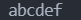``````import os
f = open( "./test/a.txt", 'a', encoding='utf-8')
f.write("abcdef")
f.close()
x = open("./test/a.txt", 'r', encoding='utf-8')